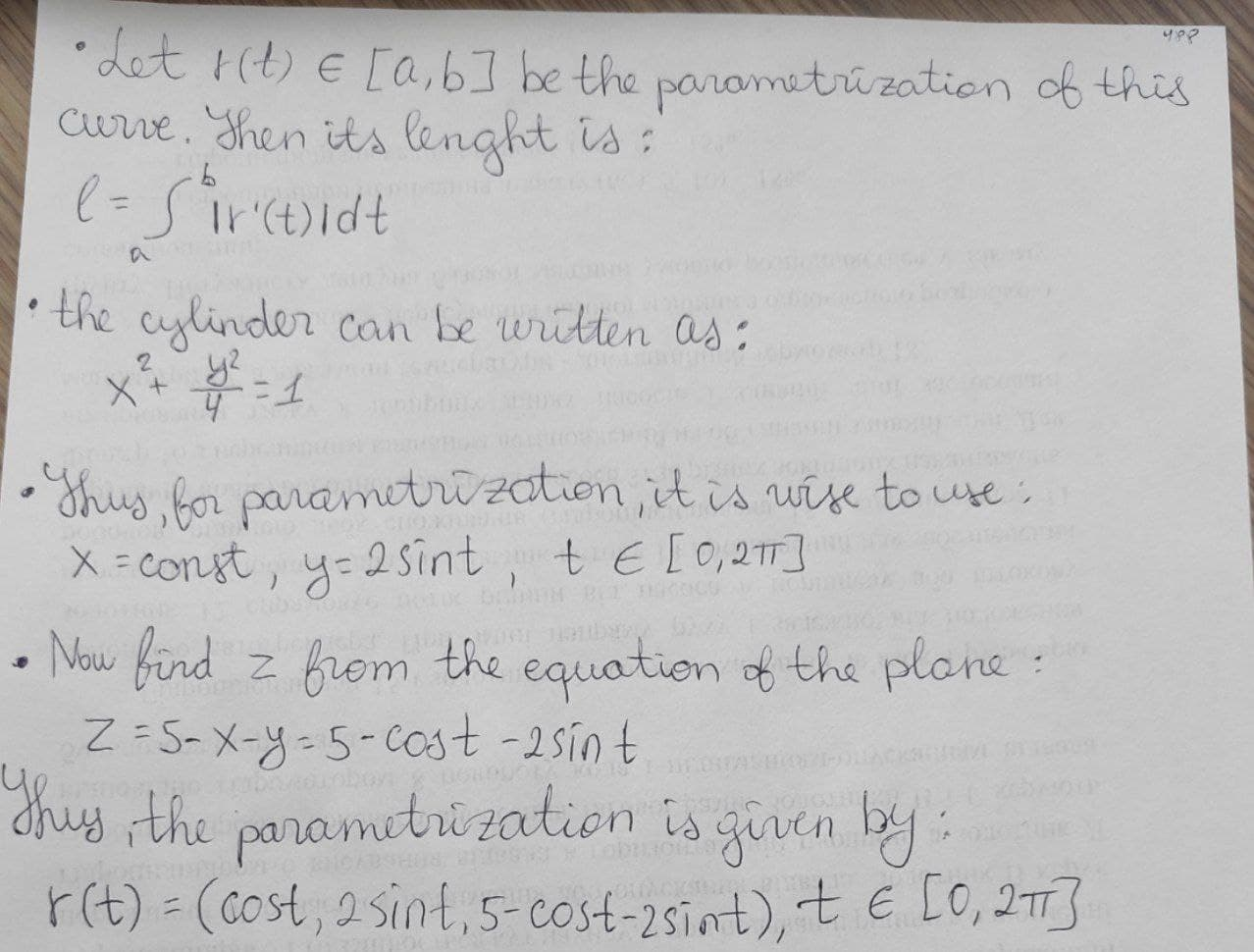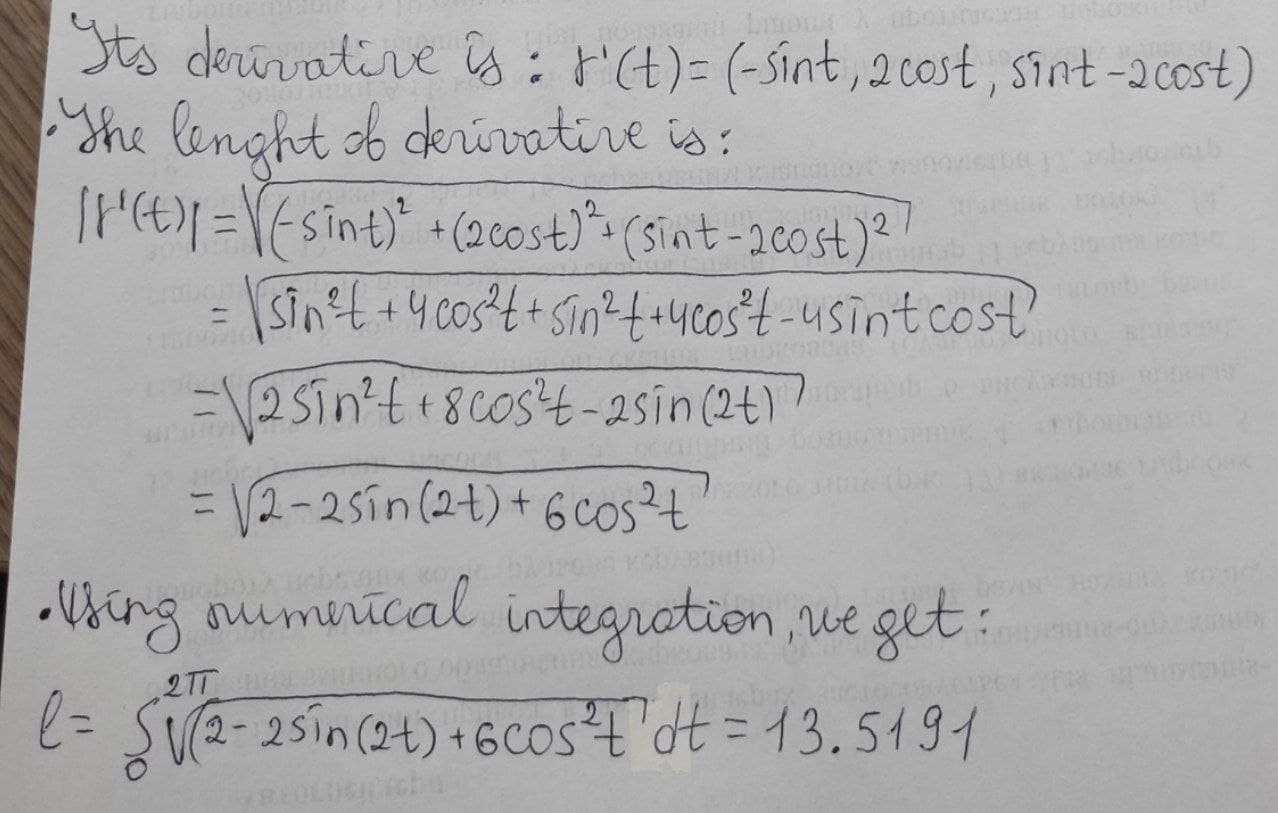# Find, correct to four decimal places, the length of the curve of intersection of the cylinder 4x^2 + y^2 = 4 and the plane x + y + z = 5.nagasenaz 2021-01-08 Answered

Find, correct to four decimal places, the length of the curve of intersection of the cylinder $4{x}^{2}+{y}^{2}=4$ and the plane $x+y+z=5.$

You can still ask an expert for help

• Questions are typically answered in as fast as 30 minutes

Solve your problem for the price of one coffee

• Math expert for every subject
• Pay only if we can solve itjlo2niT
I think this example is solved like this: